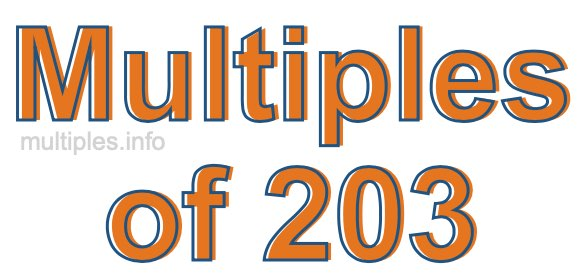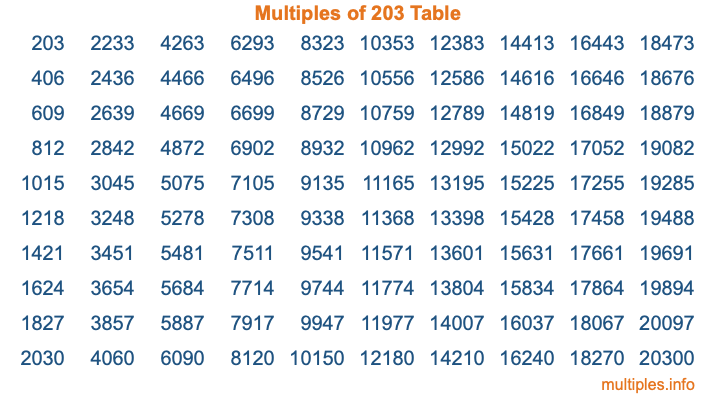Multiples of 203Welcome to the Multiples of 203 page. Here we will first teach you everything you will ever need to know about the multiples of 203, and then give you a study guide summary of everything we taught you to make sure you remember it all. Use this page to look up facts and learn information about the multiples of 203. This page will make you a multiples of two hundred three expert!

Definition of Multiples of 203
Multiples of 203 are all the numbers that when divided by 203 equal an integer. Each of the multiples of 203 are called a multiple. A multiple of 203 is created by multiplying 203 by an integer.

Therefore, to create a list of multiples of 203, you start with 1 multiplied by 203, then 2 multiplied by 203, then 3 multiplied by 203, and so on for as long as you want. Thus, the list of the first five multiples of 203 is 203, 406, 609, 812, and 1015. To see a larger list of multiples of 203, see the printable image of Multiples of 203 further down on this page. We also have a category where you can choose any nth multiple of 203.

Multiples of 203 Checker
The Multiples of 203 Checker below checks to see if any number of your choice is a multiple of 203. In other words, it checks to see if there is any number (integer) that when multiplied by 203 will equal your number. To do that, we divide your number by 203. If the the quotient is an integer, then your number is a multiple of 203.

Is  a multiple of 203?

Least Common Multiple of 203 and ...
A Least Common Multiple (LCM) is the lowest multiple that two or more numbers have in common. This is also called the smallest common multiple or lowest common multiple and is useful to know when you are adding our subtracting fractions. Enter one or more numbers below (203 is already entered) to find the LCM.

Check out our LCM Calculator if you need more details about the Least Common Multiple or if you need the LCM for different numbers for adding and subtraction fractions.

nth Multiple of 203
As we stated above, 203 is the first multiple of 203, 406 is the second multiple of 203, 609 is the third multiple of 203, and so on. Enter a number below to find the nth multiple of 203.

th multiple of 203

Multiples of 203 vs Factors of 203
203 is a multiple of 203 and a factor of 203, but that is where the similarities end. All postive multiples of 203 are 203 or greater than 203. All positive factors of 203 are 203 or less than 203.

Below is the beginning list of multiples of 203 and the factors of 203 so you can compare:

Multiples of 203: 203, 406, 609, 812, 1015, etc.

Factors of 203: 1, 7, 29, 203

As you can see, the multiples of 203 are all the numbers that you can divide by 203 to get a whole number. The factors of 203, on the other hand, are all the whole numbers that you can multiply by another whole number to get 203.

It's also interesting to note that if a number (x) is a factor of 203, then 203 will also be a multiple of that number (x).

Multiples of 203 vs Divisors of 203
The divisors of 203 are all the integers that 203 can be divided by evenly. Below is a list of the divisors of 203.

Divisors of 203: 1, 7, 29, 203

The interesting thing to note here is that if you take any multiple of 203 and divide it by a divisor of 203, you will see that the quotient is an integer.

Multiples of 203 Table
Below is an image of the first 100 multiples of 203 in a table. The table is in chronological order, column by column. The first column has the first ten multiples of 203, the second column has the next ten multiples of 203, and so on.The Multiples of 203 Table is also referred to as the 203 Times Table or Times Table of 203. You are welcome to print out our table for your studies.

Negative Multiples of 203
Although not often discussed or needed in math, it is worth mentioning that you can make a list of negative multiples of 203 by multiplying 203 by -1, then by -2, then by -3, and so on, to get the following list of negative multiples of 203:

-203, -406, -609, -812, -1015, etc.

Multiples of 203 Summary
Below is a summary of important Multiples of 203 facts that we have discussed on this page. To retain the knowledge on this page, we recommend that you read through the summary and explain to yourself or a study partner why they hold true.

There are an infinite number of multiples of 203.

A multiple of 203 divided by 203 will equal a whole number.

203 divided by a factor of 203 equals a divisor of 203.

The nth multiple of 203 is n times 203.

The largest factor of 203 is equal to the first positive multiple of 203.

203 is a multiple of every factor of 203.

203 is a multiple of 203.

A multiple of 203 divided by a divisor of 203 equals an integer.

203 divided by a divisor of 203 equals a factor of 203.

Any integer times 203 will equal a multiple of 203.

Multiples of a Number
Here you can get the multiples of another number, all with the same attention to detail as we did for multiples of 203 on this page.

Multiples of
Multiples of 204
Did you find our page about multiples of two hundred three educational? Do you want more knowledge? Check out the multiples of the next number on our list!